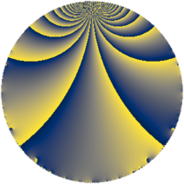# Properties

 Label 165.2.cLevel $165$ Weight $2$ Character orbit 165.c Rep. character $\chi_{165}(34,\cdot)$ Character field $\Q$ Dimension $12$ Newform subspaces $2$ Sturm bound $48$ Trace bound $4$

# Related objects

## Defining parameters

 Level: $$N$$ $$=$$ $$165 = 3 \cdot 5 \cdot 11$$ Weight: $$k$$ $$=$$ $$2$$ Character orbit: $$[\chi]$$ $$=$$ 165.c (of order $$2$$ and degree $$1$$) Character conductor: $$\operatorname{cond}(\chi)$$ $$=$$ $$5$$ Character field: $$\Q$$ Newform subspaces: $$2$$ Sturm bound: $$48$$ Trace bound: $$4$$ Distinguishing $$T_p$$: $$2$$

## Dimensions

The following table gives the dimensions of various subspaces of $$M_{2}(165, [\chi])$$.

Total New Old
Modular forms 28 12 16
Cusp forms 20 12 8
Eisenstein series 8 0 8

## Trace form

 $$12 q - 12 q^{4} + 4 q^{5} - 4 q^{6} - 12 q^{9} + O(q^{10})$$ $$12 q - 12 q^{4} + 4 q^{5} - 4 q^{6} - 12 q^{9} + 12 q^{10} - 16 q^{14} + 4 q^{16} + 16 q^{19} + 4 q^{20} - 8 q^{21} + 12 q^{24} - 4 q^{25} - 24 q^{26} + 16 q^{30} - 16 q^{34} + 12 q^{36} + 8 q^{39} - 28 q^{40} + 8 q^{44} - 4 q^{45} + 32 q^{46} - 12 q^{49} + 8 q^{50} - 8 q^{51} + 4 q^{54} + 96 q^{56} - 8 q^{60} - 40 q^{61} - 44 q^{64} + 40 q^{65} + 8 q^{66} - 16 q^{70} - 48 q^{71} + 32 q^{74} - 64 q^{76} + 32 q^{79} - 52 q^{80} + 12 q^{81} + 40 q^{85} - 16 q^{86} - 40 q^{89} - 12 q^{90} + 48 q^{94} - 48 q^{95} - 28 q^{96} + O(q^{100})$$

## Decomposition of $$S_{2}^{\mathrm{new}}(165, [\chi])$$ into newform subspaces

Label Dim $A$ Field CM Traces $q$-expansion
$a_{2}$ $a_{3}$ $a_{5}$ $a_{7}$
165.2.c.a $6$ $1.318$ 6.0.350464.1 None $$0$$ $$0$$ $$2$$ $$0$$ $$q+(\beta _{3}-\beta _{5})q^{2}+\beta _{3}q^{3}+(-2-\beta _{1}+\cdots)q^{4}+\cdots$$
165.2.c.b $6$ $1.318$ 6.0.350464.1 None $$0$$ $$0$$ $$2$$ $$0$$ $$q-\beta _{4}q^{2}+\beta _{3}q^{3}+(-\beta _{1}+\beta _{2})q^{4}+\cdots$$

## Decomposition of $$S_{2}^{\mathrm{old}}(165, [\chi])$$ into lower level spaces

$$S_{2}^{\mathrm{old}}(165, [\chi]) \cong$$ $$S_{2}^{\mathrm{new}}(55, [\chi])$$$$^{\oplus 2}$$